Subsections

# 3.2: A Doubly-Linked List

A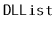(doubly-linked list) is very similar to anexcept that each nodein ahas references to both the nodethat follows it and the node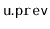that precedes it.

```  struct Node {
T x;
Node *prev, *next;
};
```

When implementing an, we saw that there were always several special cases to worry about. For example, removing the last element from anor adding an element to an empty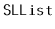requires care to ensure thatand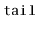are correctly updated. In a, the number of these special cases increases considerably. Perhaps the cleanest way to take care of all these special cases in ais to introduce anode. This is a node that does not contain any data, but acts as a placeholder so that there are no special nodes; every node has both aand a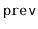, withacting as the node that follows the last node in the list and that precedes the first node in the list. In this way, the nodes of the list are (doubly-)linked into a cycle, as illustrated in Figure 3.2.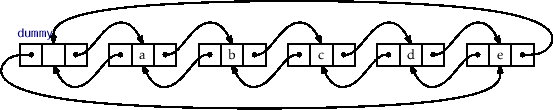```  Node dummy;
int n;
DLList() {
dummy.next = &dummy;
dummy.prev = &dummy;
n = 0;
}
```

Finding the node with a particular index in ais easy; we can either start at the head of the list (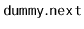) and work forward, or start at the tail of the list () and work backward. This allows us to reach theth node in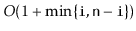time:

```  Node* getNode(int i) {
Node* p;
if (i < n / 2) {
p = dummy.next;
for (int j = 0; j < i; j++)
p = p->next;
} else {
p = &dummy;
for (int j = n; j > i; j--)
p = p->prev;
}
return (p);
}
```

The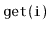andoperations are now also easy. We first find theth node and then get or set itsvalue:

```  T get(int i) {
return getNode(i)->x;
}
T set(int i, T x) {
Node* u = getNode(i);
T y = u->x;
u->x = x;
return y;
}
```

The running time of these operations is dominated by the time it takes to find theth node, and is therefore.

If we have a reference to a nodein aand we want to insert a node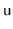before, then this is just a matter of setting,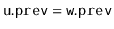, and then adjusting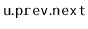and. (See Figure 3.3.) Thanks to the dummy node, there is no need to worry aboutornot existing.

```  Node* addBefore(Node *w, T x) {
Node *u = new Node;
u->x = x;
u->prev = w->prev;
u->next = w;
u->next->prev = u;
u->prev->next = u;
n++;
return u;
}
```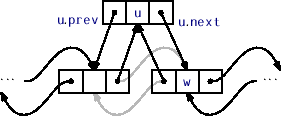Now, the list operation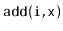is trivial to implement. We find the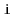th node in theand insert a new nodethat containsjust before it.

```  void add(int i, T x) {
}
```

The only non-constant part of the running time ofis the time it takes to find theth node (using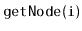). Thus,runs intime.

Removing a node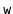from ais easy. We only need to adjust pointers at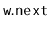andso that they skip over. Again, the use of the dummy node eliminates the need to consider any special cases:

```  void remove(Node *w) {
w->prev->next = w->next;
w->next->prev = w->prev;
delete w;
n--;
}
```

Now theoperation is trivial. We find the node with indexand remove it:

```  T remove(int i) {
Node *w = getNode(i);
T x = w->x;
remove(w);
return x;
}
```

Again, the only expensive part of this operation is finding theth node using, so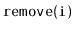runs intime.

## 3.2.2 Summary

The following theorem summarizes the performance of a:

Theorem 3..2   Aimplements the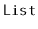interface. In this implementation, the,,andoperations run intime per operation.

It is worth noting that, if we ignore the cost of theoperation, then all operations on atake constant time. Thus, the only expensive part of operations on ais finding the relevant node. Once we have the relevant node, adding, removing, or accessing the data at that node takes only constant time.

This is in sharp contrast to the array-basedimplementations of Chapter 2; in those implementations, the relevant array item can be found in constant time. However, addition or removal requires shifting elements in the array and, in general, takes non-constant time.

For this reason, linked list structures are well-suited to applications where references to list nodes can be obtained through external means. For example, pointers to the nodes of a linked list could be stored in a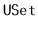. Then, to remove an itemfrom the linked list, the node that containscan be found quickly using theand the node can be removed from the list in constant time.

opendatastructures.org